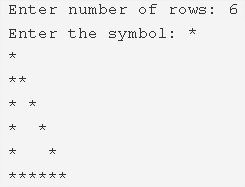# C Program Hollow Right Triangle Star Pattern

C program to print a hollow right triangle star pattern – In this article, we will discuss the multiple methods to print a hollow right triangle star pattern.

Suitable examples and sample programs have also been added so that you can understand the whole thing very clearly. The compiler has also been added with which you can execute it yourself.

The methods used to do so in this piece are as follows:

• Using For Loop
• Using While Loop
• Using Do-While Loop

As the name of the headline suggests, in this section, a right triangle is a triangle(a two-dimensional three-sided figure) with a single angle being 90 degrees.As you can see, firstly, you need to enter the number of rows. Relying on that, you will be able to print the hollow right triangle with that particular height.

Thus, the number of ways you can print the same in C programming is as follows:

## Using For Loop

1. Read the entered number of rows and store it in the variable n, read the entered character and store it into the variable ch which is a character variable.

2) The outer for loop iterates through rows from i=1 to n with the structure for(i=1,i<=n;i++).

3) The 1st inner for loop iterates through columns with the structure  for(j=1;j<=i;j++).

a) For i=1 or n(1st row,nth row),it prints symbol.

4) For other than 1’st row, nth row, the 2nd inner for loop for(j=1;j<=i;j++) iterates through columns.

a) Prints symbol if j=1 or i.

b) Prints space if j!=1 or j!=i.

5) Cursor comes to next line for each iteration of outer for loop.

Output:

### Using While Loop

1. The outer while loop iterates through rows until the condition i<=n becomes false. If the condition fails then it terminates the loop.

2) If i=1 or n,

The 1st inner while loop iterates through columns until the condition j<=i becomes false.

Prints symbol and j value increased by 1.

3) If i!=1 or i!=n,

The 2nd inner while loop iterates through columns until the condition j<=i becomes false.

Prints symbol if j=1 or i otherwise it prints space.

j value increased by 1.

4) Cursor comes to the next line for each iteration of the outer while loop.

5) i value increased by 1.

Output:

#### Using Do-While Loop

1. The outer do-while loop iterates through rows.

2) If i=1 or n

The 1st inner do-while loop iterates through columns.

Prints one symbol.j value increased by i.

Checks the condition ++j<=i. If the condition is true then loop iterates again. Repeats until the condition becomes false.

3) If i!=1 or i!=n

The 2nd inner do-while loop iterates through columns.

If j=1 or i it prints symbol, otherwise it prints space.

Checks the condition ++j<=i.

4) The cursor comes to next line for each iteration of the outer do-while loop.

5) i value increased by 1.

6) Checks the condition i<=n, if the condition is true the outer loop iterates again.Repeats until the condition becomes false.

Output:
x

## C Program To Print Number Of Days In A Month | Java Tutoring

C program to input the month number and print the number of days in that ...